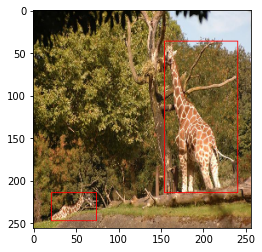# Empty output from model

Hi, I’m trying to do an object detection on a custom dataset following this tutorial

The data I was using was the coco128 dataset
The parsing into PyTorch dataset seem to be fine
Here was the “__getitem __”

``````# try printing out the image
train_img_dataset = ImageDataset(train_images, train_df, data_transforms['train'])
img, labels = train_img_dataset
sample2 = img * 255
sample2 = draw_bounding_boxes(sample2.type(torch.uint8), labels['boxes'], width=1, colors="red")
sample2 = sample2.permute(1, 2, 0).cpu().numpy()
plt.imshow(sample2)
``````non-normalized image

Here’s how I define the model

``````# load Faster RCNN pre-trained model
model = torchvision.models.detection.fasterrcnn_resnet50_fpn(pretrained=True)

# get the number of input features
# define a new head for the detector with required number of classes
``````

and here’s the output testing

``````images, targets = next(iter(train_dataloader))
images = list(image for image in images)
targets = [{k: v for k, v in t.items()} for t in targets]
output = model(images,targets)
### testing output
model.eval()
x = [images]
predictions = model(x)           # Returns predictions
predictions
``````

here’s the actual output

``````[{'boxes': tensor([], size=(0, 4), grad_fn=<StackBackward0>),
'labels': tensor([], dtype=torch.int64),
``````

I also try running for more epoch (n=10)
and here’s the result

``````Epoch:   [ 0/32]  eta: 0:00:39  lr: 0.000050  loss: 1.9125 (1.9125)  loss_classifier: 0.7819 (0.7819)  loss_box_reg: 0.3979 (0.3979)  loss_objectness: 0.5246 (0.5246)  loss_rpn_box_reg: 0.2081 (0.2081)  time: 1.2340  data: 0.1330  max mem: 4929
Epoch:   [10/32]  eta: 0:00:26  lr: 0.000050  loss: 1.3735 (1.2578)  loss_classifier: 0.4221 (0.4543)  loss_box_reg: 0.2047 (0.2168)  loss_objectness: 0.4142 (0.4064)  loss_rpn_box_reg: 0.1941 (0.1803)  time: 1.2068  data: 0.1252  max mem: 4929
Epoch:   [20/32]  eta: 0:00:14  lr: 0.000050  loss: 0.9444 (1.1087)  loss_classifier: 0.3734 (0.4063)  loss_box_reg: 0.1628 (0.1974)  loss_objectness: 0.2991 (0.3512)  loss_rpn_box_reg: 0.1051 (0.1538)  time: 1.2069  data: 0.1179  max mem: 4929
Epoch:   [30/32]  eta: 0:00:02  lr: 0.000050  loss: 0.8016 (1.0508)  loss_classifier: 0.3081 (0.3840)  loss_box_reg: 0.1539 (0.1798)  loss_objectness: 0.2436 (0.3475)  loss_rpn_box_reg: 0.0634 (0.1394)  time: 1.2258  data: 0.1177  max mem: 4929
Epoch:   [31/32]  eta: 0:00:01  lr: 0.000050  loss: 0.8016 (1.0543)  loss_classifier: 0.3081 (0.3855)  loss_box_reg: 0.1539 (0.1817)  loss_objectness: 0.2436 (0.3480)  loss_rpn_box_reg: 0.0634 (0.1390)  time: 1.2204  data: 0.1168  max mem: 4929
Epoch:  Total time: 0:00:38 (1.2164 s / it)
creating index...
index created!
Test:  [ 0/32]  eta: 0:00:22  model_time: 0.5850 (0.5850)  evaluator_time: 0.0050 (0.0050)  time: 0.7020  data: 0.1070  max mem: 4929
Test:  [31/32]  eta: 0:00:00  model_time: 0.4090 (0.3911)  evaluator_time: 0.0080 (0.0103)  time: 0.5440  data: 0.1069  max mem: 4929
Test: Total time: 0:00:16 (0.5123 s / it)
Averaged stats: model_time: 0.4090 (0.3911)  evaluator_time: 0.0080 (0.0103)
Accumulating evaluation results...
DONE (t=0.37s).
IoU metric: bbox
Average Precision  (AP) @[ IoU=0.50:0.95 | area=   all | maxDets=100 ] = 0.000
Average Precision  (AP) @[ IoU=0.50      | area=   all | maxDets=100 ] = 0.000
Average Precision  (AP) @[ IoU=0.75      | area=   all | maxDets=100 ] = 0.000
Average Precision  (AP) @[ IoU=0.50:0.95 | area= small | maxDets=100 ] = 0.000
Average Precision  (AP) @[ IoU=0.50:0.95 | area=medium | maxDets=100 ] = 0.000
Average Precision  (AP) @[ IoU=0.50:0.95 | area= large | maxDets=100 ] = 0.000
Average Recall     (AR) @[ IoU=0.50:0.95 | area=   all | maxDets=  1 ] = 0.000
Average Recall     (AR) @[ IoU=0.50:0.95 | area=   all | maxDets= 10 ] = 0.000
Average Recall     (AR) @[ IoU=0.50:0.95 | area=   all | maxDets=100 ] = 0.000
Average Recall     (AR) @[ IoU=0.50:0.95 | area= small | maxDets=100 ] = 0.000
Average Recall     (AR) @[ IoU=0.50:0.95 | area=medium | maxDets=100 ] = 0.000
Average Recall     (AR) @[ IoU=0.50:0.95 | area= large | maxDets=100 ] = 0.002
``````

I’m not quite sure why the model isn’t predicting anything. The loss seems to be decreasing (not sure what’s the appropriate number for losses). Should I try running for more epochs?

No, it shouldn’t be a case of training for more epochs. Even after 10 epochs you should not be getting all the stats to be 0.000.

Did you make any changes to the code? What if you try running the tutorial directly in google Colab or running the source code provided, do you still see the same results?

The only change I can think of that might cause problem was the way I do transformation.
Here’s how I tried doing it (using albumentations )

``````import albumentations as A
from albumentations.pytorch import ToTensorV2

def get_train_transform(box_format="yolo", to_img_size = 640):
return A.Compose([
A.Resize(to_img_size, to_img_size),
A.Normalize(mean=(0.485, 0.456, 0.406), std=(0.229, 0.224, 0.225)),
ToTensorV2(p=1.0),
], bbox_params=A.BboxParams(format=box_format, label_fields=["labels"]))

# define the validation transforms
def get_valid_transform(box_format="yolo", to_img_size = 640):
return A.Compose([
A.Resize(to_img_size, to_img_size),
A.Normalize(mean=(0.485, 0.456, 0.406), std=(0.229, 0.224, 0.225)),
ToTensorV2(p=1.0),
], bbox_params={'format': box_format, 'label_fields': ['labels']})
``````

I tried running the tutorial code as is and it seems to work fine.
I did modified the dataset class quite bit but printing them out seems fine to me.